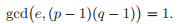# Let p and q be distinct primes and let e and d be integers satisfying de ≡ 1 (mod (p −… 1 answer below »

Let p and q be distinct primes and let e and d be integers satisfying

de ≡ 1 (mod (p − 1)(q − 1)).

Suppose further that c is an integer with gcd(c, pq) > 1. Prove that x ≡ cd (mod pq) is a solution to the congruence xe ≡ c (mod pq), thereby completing the proof of Proposition 3.4.

Proposition 3.4

Let p and q be distinct primes and let e ≥ 1 satisfy